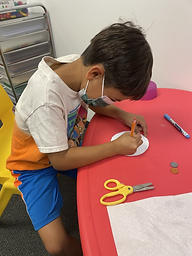## Ms. Caitlyn

### Target 1​

###### Lesson Type:

Continuation

Number Operation

:

Fractional Numbers

Identify US currency coins.

###### 1:

Visually distinguish between a dime and penny.

###### 2:

Become familiar with both the front and back sides of dimes and pennies.

###### 3:

Know the value of dimes and pennies.

1st

###### Vocabulary:

Dime, Penny, Size, Color, Front, Back

Activities:

Students created their own dimes and pennies.

Students were encouraged to not only distinguish the colors of dimes and pennies, but the size and image differences.### Home Exploration

###### Guiding Questions:## Absent Students:

### Target 2

:

###### 1:

Use currency symbols (\$ and ₵) appropriately.

###### 2:

Develop strategies for counting currency easily, count the dimes first (skip counting by tens) and then the pennies (counting on by ones).

###### 3:

Accurately count a set of money.

1st

###### Vocabulary:

Dime, Penny, Cents, Skip Count, Add

Activities:

Students were given a set of paper strips. Each paper strip had either a collection of dimes and pennies, or an item with a price. Students had to count the dimes and pennies and match them to the corresponding price.### Home Exploration

###### Guiding Questions:### Target 3

:

###### 1:

Recognize the arithmetic symbols in a subtraction equation: minus (-) and equals (=).

###### 2:

Understand that subtraction means to take a quantity away from another quantity.

###### 3:

Understand that subtracting numbers means making a smaller number.

###### 4:

Understand that subtraction equals can be written both vertically and horizontally.

###### 5:

Understand that the same process is utilized to add three or more whole numbers that is used to add only two numbers.

2nd

###### Vocabulary:

Subtract, Difference, Minus

Activities:

Students colored subtraction color-by-number worksheets. They drew dots on their whiteboards and erased to solve.### Home Exploration